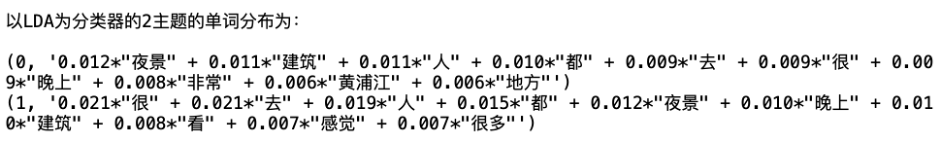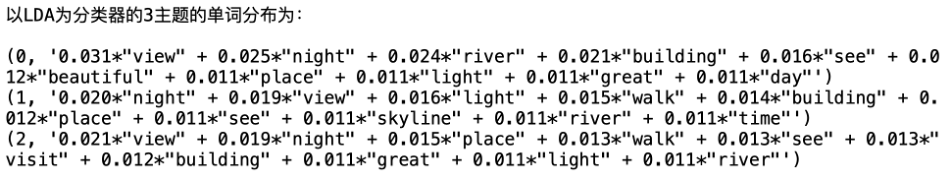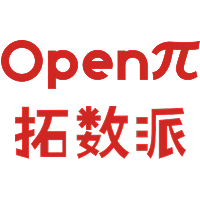# 数据科学在文本分析中的应用 ：中英文 NLP（下）2023-03-27

## 数据建模

• 判定语料是否为目标语言
• 分离并分析语料中的 emoji
• 根据语言类型（中文或英文）对语料进行清理
• 使用语言模型进行分词，并计算词频
• 使用语言模型进行情感分析
• 主题模型进行文本分类

### 1.清理非目标语言

```def filter_text_lan(s,lan):
if lan == 'zh':
s_lst = re.split('。',s.replace('.','。'))
else:
s_lst = s.split('. ')
s_new = ''
for sentence in s_lst:
s_lan = langid.classify(sentence)
if s_lan == lan:
append_s = sentence+'. '
s_new += append_s
return s_new
```

```df['comment_text'] = df['title'] + '。' + df['content']
df['text_zh_filtered'] = df['comment_text'].apply(lambda x: filter_text_lan(x,"zh"))
```

```df['comment_text'] = df['title'] + '. ' + df['content']
df['text_en_filtered'] = df['comment_text'].apply(lambda x: filter_text_lan(x,"en"))
```

### 2.处理 emoji 并清理语料中不需要的内容

Emoji 作为深受全球喜爱的表情符号，在本项目的语料中也频繁出现。为了后续分词等建模步骤，我们必须先将 emoji 从文本中分离出来，结果传送至数据库中。

```def extract_emojies(s):
emoji_list = []
for c in s:
if c in emoji.EMOJI_DATA:
emoji_list.append(c)
return list(dict.fromkeys(emoji_list))
```

```emojis_series = df['text_zh_filtered'].apply(extract_emojies)
```

```mlb = MultiLabelBinarizer()
emojis_series_transformed = pd.DataFrame(mlb.fit_transform(emojis_series),
columns=mlb.classes_,
index=emojis_series.index)
```

```df_transformed = df.join(emojis_series_transformed)
```

```df_transformed.columns
```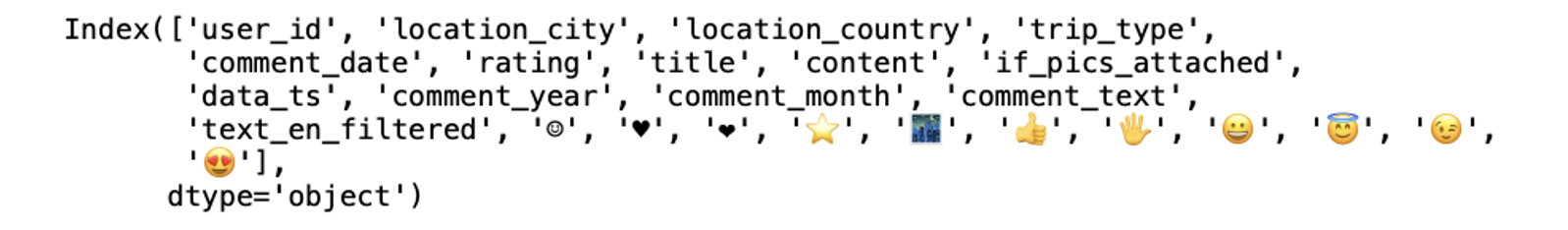```def remove_emoji(s):
s = demoji.replace(s,'')
return s.strip()
```

```def remove_zh_chars(s):
if "阅读更多" in s:
s = s.replace("\n阅读更多。", '')
s = demoji.replace(s,'')
return s.strip()
```

```df_transformed['text_en_cleaned'] = df_transformed['text_en_filtered'].apply(remove_emoji)
```

```df_transformed['text_zh_cleaned'] = df_transformed['text_zh_filtered'].apply(remove_zh_chars)
```

### 3.情感分析（中文文本）

```random.seed(1024)
sample_text = sample(list(df_transformed['text_zh_cleaned']),k=20)
```

```marked_sentiment = [1, 0, 1, 1, 0, 1, 1, 1, 1, 1, 1, 1, 1, 1, 1, 1, 1, 1, 1, 1]
```

```# SnowNLP
def get_snownlp_senti(s):
snownlp_obj = SnowNLP(s)
s_score = snownlp_obj.sentiments
if s_score > 0.5:
return 1
else:
return 0
# Cemotion
c = Cemotion()
def get_cemotion_senti(s,model=c):
score = model.predict(s)
if score > 0.5:
return 1
else:
return 0
snownlp_result = list(map(get_snownlp_senti, sample_text))
cemotion_result = list(map(get_cemotion_senti, sample_text))
```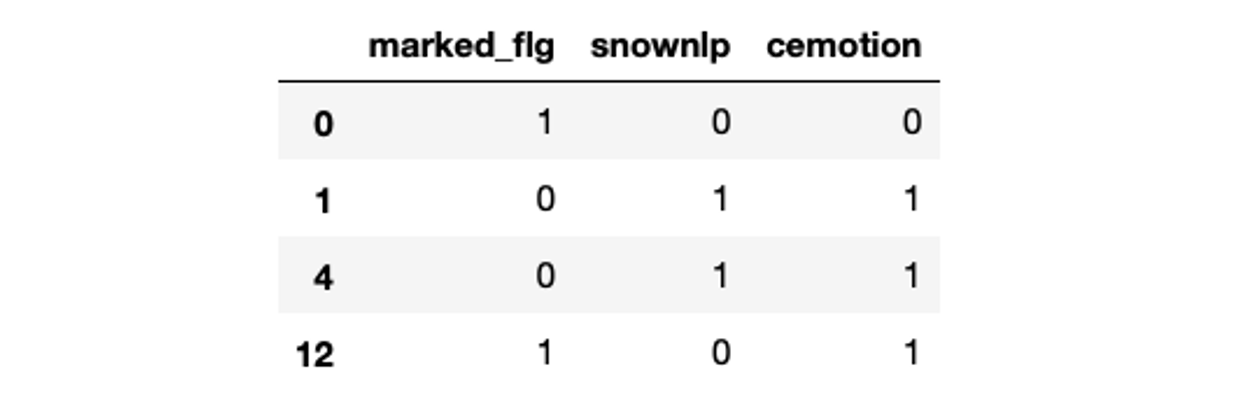SnowNLP 标出了以下文字为负向情绪：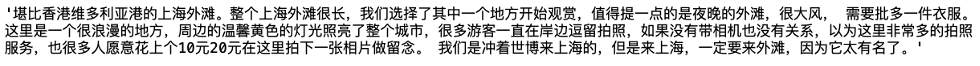```def get_sentiment_score(data, text_col="text_zh_cleaned"):
data_cp = data.copy()
text_series = data_cp[text_col]
## SnowNLP
#snownlp_result = list(map(get_snownlp_senti, text_series.to_list()))
#data_cp['snownlp_senti'] = snownlp_result
## Cemotion
cemotion_result = list(map(get_cemotion_senti, text_series.to_list()))
data_cp['cemotion_senti'] = cemotion_result
return data_cp
```### 4.情感分析（英文文本）

```def get_en_senti(s_text):
score = TextBlob(s_text).sentiment
if score > 0:
return 1
else:
return 0
```

```df_transformed['blob_senti'] = df_transformed['text_en_cleaned'].apply(get_en_senti)
```

```# 挑选需要的变量
cols_to_db = ['user_id', 'location', 'trip_type', 'comment_date', 'comment_year', 'comment_month',
'rating', 'if_pics_attached', 'data_ts','text_zh_cleaned','cemotion_senti',
'☔', '⛈', '🈶', '🌟', '🍃', '🍵',
'🎬', '🏻', '👍', '👼','💖', '😁', '😂', '😊', '😌', '😍', '😏', '🤗', '🥁']
```

```# 将emoji转化为英文字符串
cols_to_db_transformed = list(map(emoji.demojize,cols_to_db))
```

```# 选取需要的数据，并更新变量名称
df_to_db = df_senti[cols_to_db]
df_to_db.columns = cols_to_db_transformed
```

```# 将数据写进数据库
df_to_db.to_sql("上海_上海_外滩_cleaned_senti_ZH", engine, if_exists="replace", index=False)
```

```cols_to_db = ['user_id', 'location_city', 'location_country', 'trip_type', 'comment_date',
'comment_year', 'comment_month',
'rating', 'if_pics_attached', 'data_ts','text_en_cleaned', 'blob_senti',
'☺', '♥', '❤', '⭐', '🌃', '👍', '🖐','😀', '😇', '😉', '😍']
cols_to_db_transformed = list(map(emoji.demojize,cols_to_db))
df_to_db = df_transformed[cols_to_db]
df_to_db.columns = cols_to_db_transformed
df_to_db.to_sql("Shanghai_Shanghai_The Bund (Wai Tan)_cleaned_senti_EN", engine, if_exists="replace", index=False)
```

### 5.基于情感倾向的 emoji 分析

```# 摘取需要的变量
emoji_count_cols = ['☔', '⛈', '🈶', '🌟', '🍃', '🍵', '🎬', '', '👍', '👼',
'💖', '😁', '😂', '😊', '😌', '😍', '😏', '🤗', '🥁','cemotion_senti']
```

```# 提取情绪倾向
emoji_only_df = df_senti[emoji_count_cols]
```

```# 将emoji转换为英文字符串
emoji_only_df.columns = list(map(emoji.demojize,emoji_count_cols))
emoji_counts = (emoji_only_df
.groupby('cemotion_senti').sum().transpose()
.reset_index()
.rename_axis(None, axis=1)
.rename(columns={'index':'emoji',0:'senti_0_count',1:'senti_1_count'})
.sort_values(by=['senti_0_count','senti_1_count'],ascending=False)
)
emoji_counts
``````emoji_count_cols = ['☺', '♥', '❤', '⭐', '🌃', '👍', '🖐','😀', '😇', '😉', '😍','blob_senti']
emoji_only_df = df_transformed[emoji_count_cols]
emoji_only_df.columns = list(map(emoji.demojize,emoji_count_cols))
emoji_counts = (emoji_only_df
.groupby('blob_senti').sum().transpose()
.reset_index()
.rename_axis(None, axis=1)
.rename(columns={'index':'emoji',0:'senti_0_count',1:'senti_1_count'})
.sort_values(by=['senti_0_count','senti_1_count'],ascending=False)
)
emoji_counts
```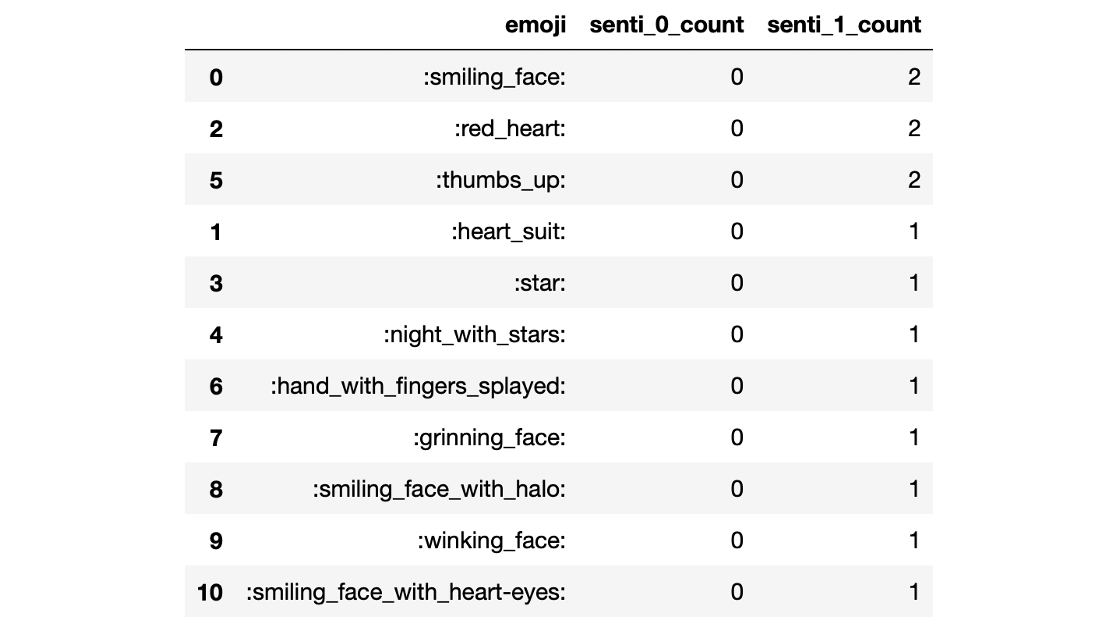```emoji_counts.to_sql("上海_上海_外滩_emoji_count_ZH", engine, if_exists="replace", index=False)
```

```emoji_counts.to_sql("Shanghai_Shanghai_The Bund (Wai Tan)_emoji_count_EN", engine, if_exists="replace", index=False)
```

### 6.分词（中文文本）

• 删除空白格和标点符号
• 使用语言对应的算法将文本字符串分词
• 检查分词结果，删除停止词

```# 选取评论
test_list = df_transformed.loc[:5,'text_zh_cleaned']
```

```# 开启paddle模式
```

```# 打印分词结果
for s in test_list:
print(s)
print("Paddle Mode: " + '/ '.join(list(seg_list)))
seg_list = jieba.cut(s, cut_all=True)
print("Full Mode: " + "/ ".join(seg_list)) # 全模式
seg_list = jieba.cut(s, cut_all=False)
print("Default Mode: " + "/ ".join(seg_list)) # 精确模式
seg_list = jieba.cut_for_search(s) # 搜索引擎模式
print("Search Mode: " + "/ ".join(seg_list))
```

```十点左右开始人比较少。感觉不论作为游客还是居民，在这里散步都是非常享受的。 黄浦江边的风很舒服，江对面灯光之下就是繁华的上海，很浪漫。
Paddle Mode: 十点/ 左右/ 开始/ 人/ 比较/ 少/ 。/ 感觉/ 不/ 论/ 作为/ 游客/ 还是/ 居民/ ，/ 在这里/ 散步/ 都是/ 非常/ 享受/ 的/ 。 / 黄浦/ 江边/ 的/ 风/ 很舒服/ ，江/ 对面/ 灯光/ 之/ 下/ 就是/ 繁华/ 的/ 上海/ ，/ 很/ 浪漫/ 。
Full Mode: 十点/ 左右/ 开始/ 人/ 比较/ 较少/ 。/ 感觉/ 不论/ 作为/ 游客/ 还是/ 居民/ ，/ 在/ 这里/ 散步/ 都/ 是非/ 非常/ 享受/ 的/ 。/ / / 黄浦/ 黄浦江/ 浦江/ 江边/ 的/ 风/ 很/ 舒服/ ，/ 江/ 对面/ 灯光/ 之下/ 就是/ 繁华/ 的/ 上海/ ，/ 很/ 浪漫/ 。
Default Mode: 十点/ 左右/ 开始/ 人/ 比较/ 少/ 。/ 感觉/ 不论/ 作为/ 游客/ 还是/ 居民/ ，/ 在/ 这里/ 散步/ 都/ 是/ 非常/ 享受/ 的/ 。/ / 黄浦江/ 边/ 的/ 风/ 很/ 舒服/ ，/ 江/ 对面/ 灯光/ 之下/ 就是/ 繁华/ 的/ 上海/ ，/ 很/ 浪漫/ 。
Search Mode: 十点/ 左右/ 开始/ 人/ 比较/ 少/ 。/ 感觉/ 不论/ 作为/ 游客/ 还是/ 居民/ ，/ 在/ 这里/ 散步/ 都/ 是/ 非常/ 享受/ 的/ 。/ / 黄浦/ 浦江/ 黄浦江/ 边/ 的/ 风/ 很/ 舒服/ ，/ 江/ 对面/ 灯光/ 之下/ 就是/ 繁华/ 的/ 上海/ ，/ 很/ 浪漫/ 。
```

```外滩夜景yyds。超级美的浦江夜景三件套～今天恋爱了 晚上吃过晚饭，去江边走一走，散散心，消消食，真的感觉超级幸福～外滩估计是每个人来上海必打卡的项目之一了。
Paddle Mode: 外滩/ 夜景/ yyds。/ 超级/ 美/ 的/ 浦江/ 夜景/ 三件套/ ～/ 今天/ 恋爱/ 了/ / 晚上/ 吃/ 过/ 晚饭/ ，/ 去/ 江边/ 走一走/ ，散散心，消消食，/ 真/ 的/ 感觉/ 超级幸福～/ 外滩/ 估计/ 是/ 每个人/ 来/ 上海/ 必/ 打卡/ 的/ 项目/ 之/ 一/ 了/ 。
Full Mode: 外滩/ 夜景/ yyds/ 。/ 超级/ 美的/ 浦江/ 夜景/ 三件/ 三件套/ 件套/ ～/ 今天/ 恋爱/ 了/ / / / 晚上/ 吃/ 过晚/ 晚饭/ ，/ 去/ 江边/ 走/ 一/ 走/ ，/ 散散/ 散散心/ 散心/ ，/ 消消/ 消食/ ，/ 真的/ 感觉/ 超级/ 幸福/ ～/ 外滩/ 估计/ 是/ 每个/ 个人/ 来/ 上海/ 必/ 打卡/ 的/ 项目/ 之一/ 了/ 。
Default Mode: 外滩/ 夜景/ yyds/ 。/ 超级/ 美的/ 浦江/ 夜景/ 三件套/ ～/ 今天/ 恋爱/ 了/ / 晚上/ 吃/ 过/ 晚饭/ ，/ 去/ 江边/ 走/ 一/ 走/ ，/ 散散心/ ，/ 消/ 消食/ ，/ 真的/ 感觉/ 超级/ 幸福/ ～/ 外滩/ 估计/ 是/ 每个/ 人来/ 上海/ 必/ 打卡/ 的/ 项目/ 之一/ 了/ 。
Search Mode: 外滩/ 夜景/ yyds/ 。/ 超级/ 美的/ 浦江/ 夜景/ 三件/ 件套/ 三件套/ ～/ 今天/ 恋爱/ 了/ / 晚上/ 吃/ 过/ 晚饭/ ，/ 去/ 江边/ 走/ 一/ 走/ ，/ 散散/ 散心/ 散散心/ ，/ 消/ 消食/ ，/ 真的/ 感觉/ 超级/ 幸福/ ～/ 外滩/ 估计/ 是/ 每个/ 人来/ 上海/ 必/ 打卡/ 的/ 项目/ 之一/ 了/ 。
```

```# 读取停止词
with open('hit_stopwords.txt','r') as f:
stopwords = [line.strip() for line in f]
# 将上海、外滩这类在语料中频繁出现但是并不需要的词加入停止词表
stopwords = stopwords+['上海','外滩']
def text_tokenizer_zh(s):
# 删除空白格
s = s.strip()
# 删除标点符号
s = re.sub(r"[0-9\s+\.\!\/_,\$%^*()?;；:-【】+\"\']+|[+——！，;:。？、~@#￥%……&*（）～]+", "", s)
seg_list = jieba.posseg.cut(s)
cut_list = [(x.word,x.flag) for x in seg_list if x.word not in stopwords]
return cut_list
```

```zh_text_tokenized = df_senti['text_zh_cleaned'].apply(text_tokenizer_zh)
df_senti['text_zh_tokenized'] = zh_text_tokenized
```

### 7.分词（英文文本）

```# 下载NLTK算法资源
```

```# 从NLTK中读取英文停止词资源
stop_words = stopwords.words('english')
stop_words+=["shanghai",'bund']
wl = WordNetLemmatizer()
def text_tokenizer_en(s):
# remove blank space
s = s.strip()
tags = pos_tag(word_tokenize(s))
tokenized_list = []
for word, tag in tags:
tag_lemma = tag.lower()
wntag = tag_lemma if tag_lemma in ['a', 'r', 'n', 'v'] else None
if word.lower() not in stop_words and word.isalpha():
if wntag is None:
lemma_word = wl.lemmatize(word.lower())
else:
lemma_word = wl.lemmatize(word.lower(),wntag)
tokenized_list.append((lemma_word,tag))
```

```en_text_tokenized = df_transformed['text_en_cleaned'].apply(text_tokenizer_en)
df_transformed['text_en_tokenized'] = en_text_tokenized
```

### 8.词性词频分析

• 名词（n）：n，nr，ns，nt，nz
• 动词（v）：v，vn
• 形容词（a）：a

• 名词（n）：NN，NNS，NNP，NNPS，FW
• 动词（v）：VB，VBD，VBG，VBN
• 形容词（a）：JJ，JJR，JJS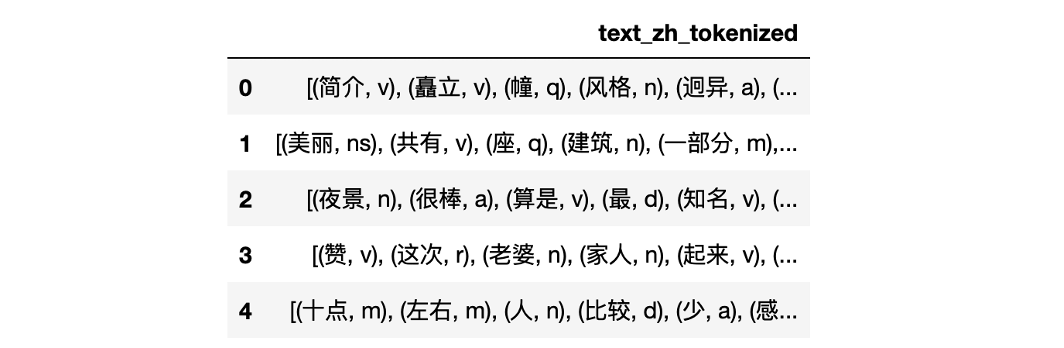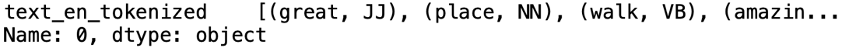```pos_map_zh = {'n':['n', 'nr', 'ns', 'nt', 'nz'], 'v':['v','vn'], 'a':['a']}
pos_map_en = {'n':['NN','NNS','NNP','NNPS','FW'], 'v':['VB','VBD','VBG','VBN'],
'a':['JJ','JJR','JJS']}
def get_word_count(token_series, pos_flg, lan):
# 将表单list中的表单元素合为单个表单
word_list = list(itertools.chain.from_iterable(token_series))
if lan.upper() == 'ZH':
pos_lst = pos_map_zh[pos_flg]
else:
pos_lst = pos_map_en[pos_flg]
word_list_filtered = [x for x in word_list if x in pos_lst]
# 计算各个元素的频率
counts = Counter(word_list_filtered)
data_items = counts.items()
data_list = list(data_items)
# 将结果转换为DataFrame输出
word_count_df = pd.DataFrame(data_list)
word_count_df.rename(columns = {0:'word', 1:'frequency'}, inplace = True)
word_count_df['pos'] = pos_flg
return word_count_df
```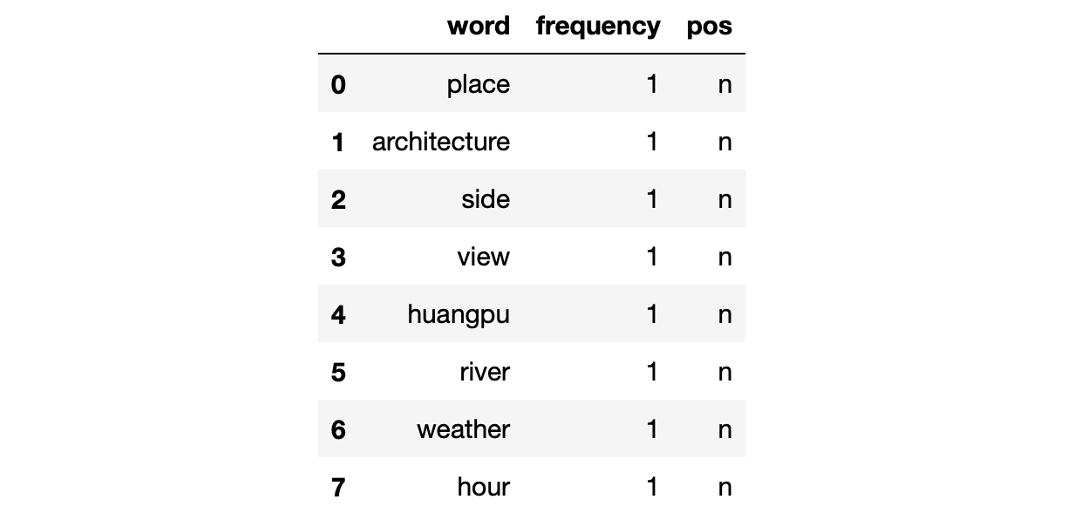```def word_count_grouped(data, token_col, lan):
data_copy = data.copy()
# 使用评论语言情绪倾向相对应的变量名
if lan.upper() == 'ZH':
senti_col = 'cemotion_senti'
pos_map = pos_map_zh
else:
senti_col = 'blob_senti'
pos_map = pos_map_en
# 计算情绪倾向，评论年份和月份变量各自去重后的值
senti_values = data[senti_col].unique()
year_values = data['comment_date'].dt.year.unique()
month_values = data['comment_date'].dt.month.unique()
result_count_df = pd.DataFrame()
# 根据特定的情绪倾向，评论年份和月份值，计算这类评论的词频
for pos_flg in pos_map.keys():
for senti in senti_values:
for y in year_values:
for m in month_values:
data_filtered = data[(data[senti_col]==senti)&(data['comment_year']==y)&(data['comment_month']==m)]
if data_filtered.shape > 0:
count_df = get_word_count(data_filtered[token_col], pos_flg, lan)
count_df[senti_col] = senti
count_df['comment_year'] = y
count_df['comment_month'] = m
result_count_df = pd.concat([result_count_df, count_df])
return result_count_df.reset_index(drop=True)
```

```word_count_df = word_count_grouped(df_senti,'text_zh_tokenized','zh')
word_count_df.to_sql("上海_上海_外滩_word_count_ZH", engine, if_exists="replace", index=False)
```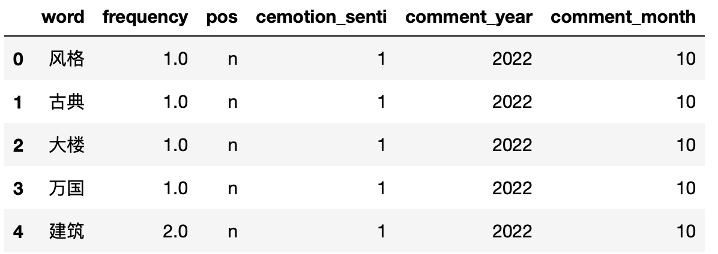```word_count_df = word_count_grouped(df_transformed,'text_en_tokenized','EN')
word_count_df.to_sql("Shanghai_Shanghai_The Bund (Wai Tan)_word_count_EN", engine, if_exists="replace", index=False)
```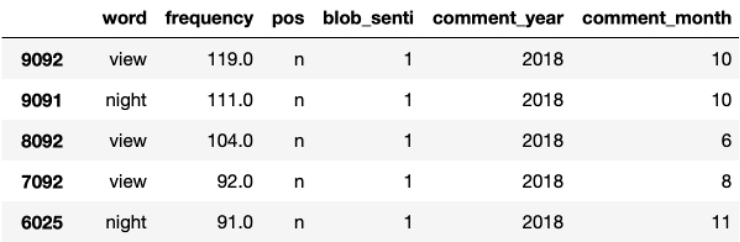### 9.关键词分析

Jieba 中包含两种更高阶的用来计算关键词的算法：TF-IDF 和 TextRank。其中 TF-IDF 作为词袋模型（Bag of Word）也经常被用来作为将文字内容数字化的算法之一。由于这两种算法自带清理模块，我们直接使用了原文本而并非去除了停用词的语料。

```s_concat_list = ''.join(df_senti['text_zh_cleaned'].to_list())
```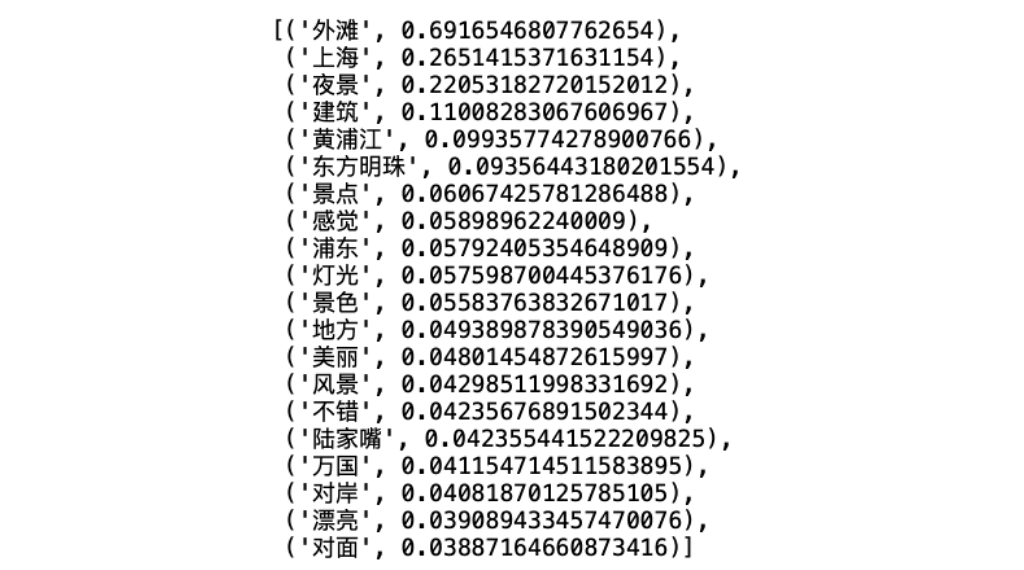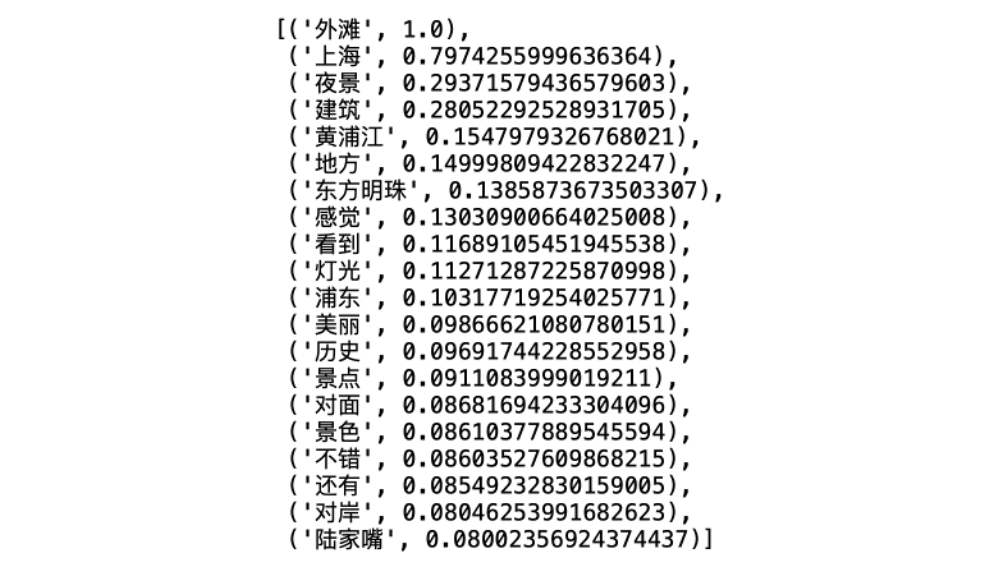### 10.词云展示

```def get_word_cloud_pos(count_df,font_pth, lan):
pos_values = count_df['pos'].unique()
fig = plt.figure(figsize=(30,25))
for i,pos_tag in enumerate(pos_values):
word_count_sum = count_df[count_df['pos']==pos_tag].groupby(['word'])[['frequency']].sum().reset_index()
word_count_dict = word_count_sum.set_index('word').to_dict()['frequency']
wc = WordCloud(width=1000, height=800,background_color = 'white',font_path = font_pth).generate_from_frequencies(word_count_dict)
ax.imshow(wc)
ax.title.set_text("Word Count for POS: "+pos_tag)
ax.axis('off')
plt.savefig('word_cloud_'+lan+'.png')
return
```

```get_word_cloud_pos(word_count_df,'ALIBABA-PUHUITI-REGULAR.TTF','ZH')
```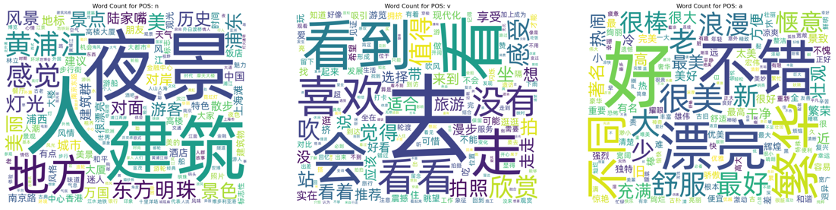```get_word_cloud_pos(word_count_df,'ALIBABA-PUHUITI-REGULAR.TTF','EN')
```### 11.主题模型文本分类

```def get_topics(data, topics_n, token_col):
# 建立分词表
l_words_list = [[word for word in doc] for doc in data[token_col]]
# 建立语料库
word_dict = corpora.Dictionary(l_words_list)
corpus = [word_dict.doc2bow(text) for text in l_words_list]
# 建立模型
lda_model = models.LdaMulticore(corpus=corpus,
id2word=word_dict,
num_topics=topics_n)
# 打印文本分类结果
topic_list_lda = lda_model.print_topics()
# topic_list_lsi = lsi.print_topics(16)
print("以LDA为分类器的"+str(topics_n)+"主题的单词分布为：\n")
for topic in topic_list_lda:
print(topic)
```